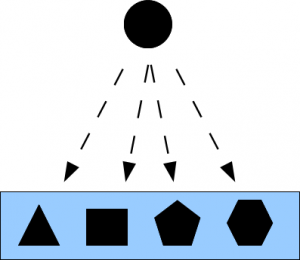# On the Objective Indefiniteness Interpretation of Quantum MechanicsThis version of the paper is the final draft of the paper which is forthcoming in Quantum Mechanics Interpretations.

Classical physics and quantum physics suggest two different meta-physical conceptions of reality: the classical notion of a objectively definite reality “all the way down,” and the quantum conception of an objectively indefinite type of reality. Part of the problem of interpreting quantum mechanics (QM) is the problem of making sense out of an objectively indefinite reality. Our sense-making strategy is to follow the math by showing that the mathematical way to describe indefiniteness is by partitions (quotient sets or equivalence relations). We follow the partitional math at both the levels of sets and complex vector spaces. We develop a pedagogical model of QM over sets or QM/sets so that the interpretive questions about QM can be split into two parts: QM/sets and full QM. The strategy is to first answer a question “in principle” in the simple setting of QM/sets, and then translate the answer into full QM. The pedagogical use of QM/sets is illustrated by modeling the double-slit experiment. But principally this approach is used in analyzing the notion of measurement. The mathematics of both QM/sets and full QM is written in the language of partitions. The reason is that partitions are the mathematical way to describe indefiniteness. In this manner, following the math suggests that the realistic or objective way to interpret quantum mechanics is, as emphasized by Abner Shimony, the objective indefiniteness interpretation